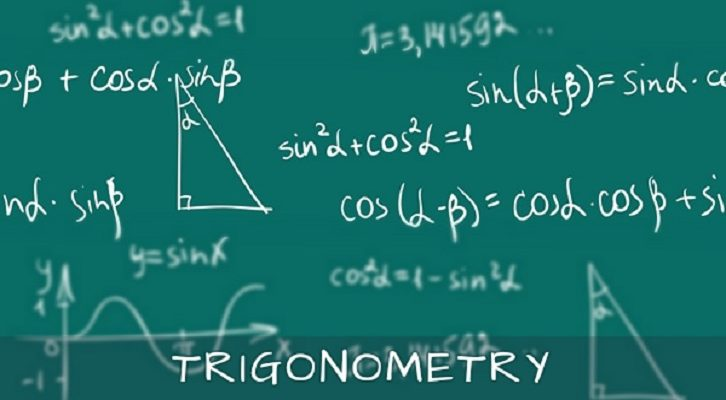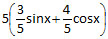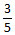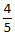# CAT – Trigonometry Questions

Question:
3sinx + 4cosx + r is always greater than or equal to 0. What is the smallest value ‘r’ can to take?
A.  5
B.  -5
C.  4
D.  3

Explanation:
3sinx + 4cosx -r-r= cosA  => sinA =5(sinx cosA + sinA cosA) -r
5(sin(x + A) -r
5sin (x + A) -r
-1 < sin (angle) <1
5sin (x + A) -5
rmin = 5
Difficulty Level 2